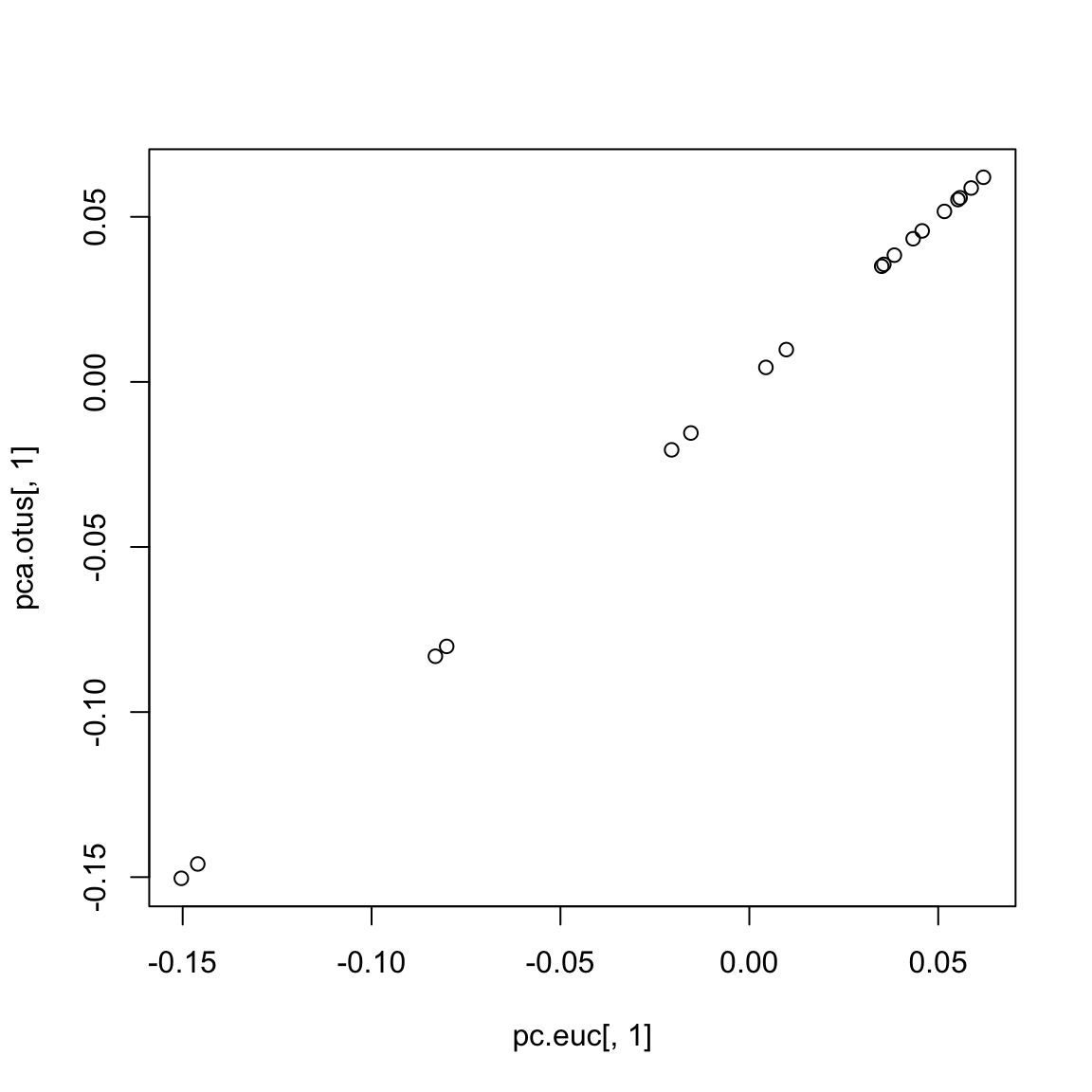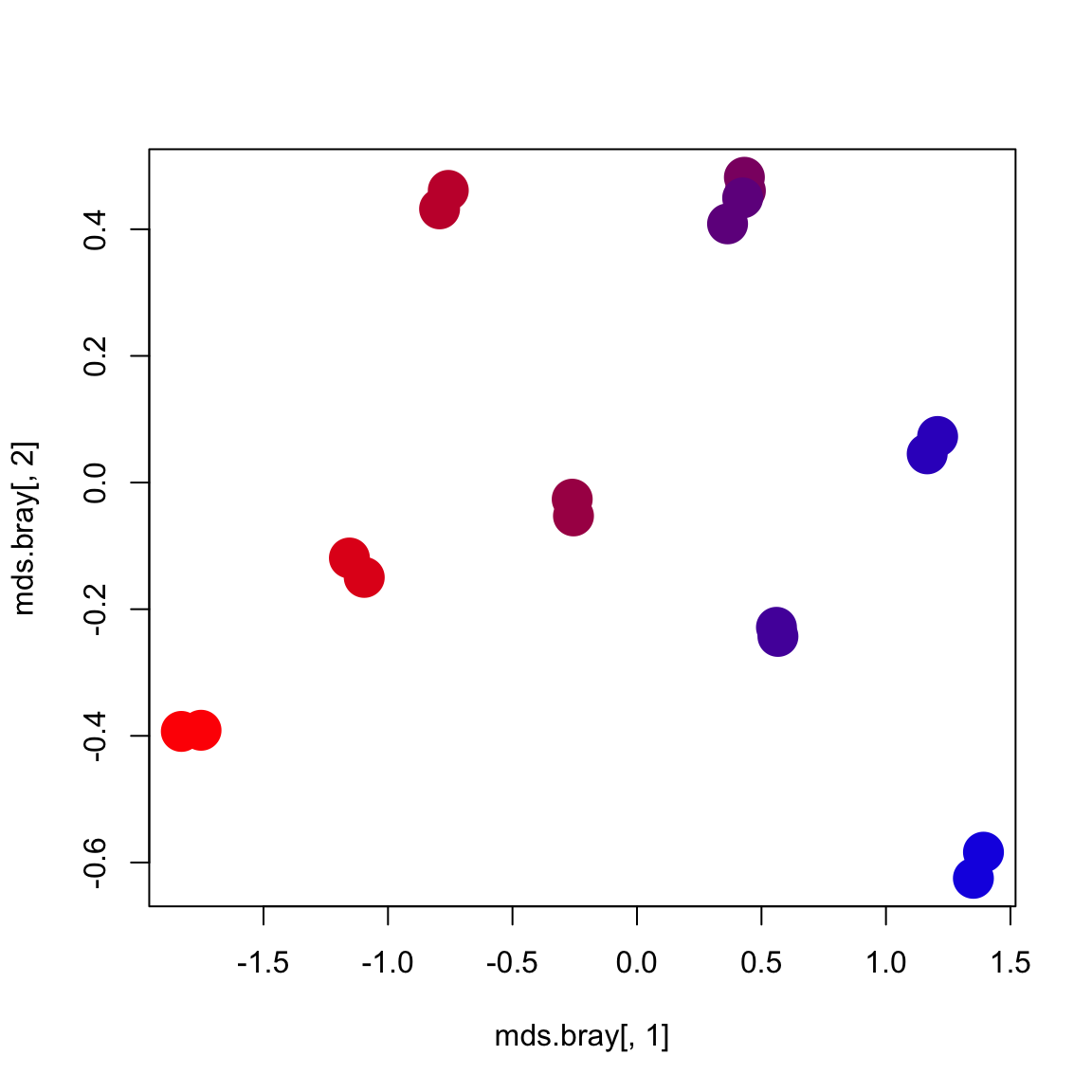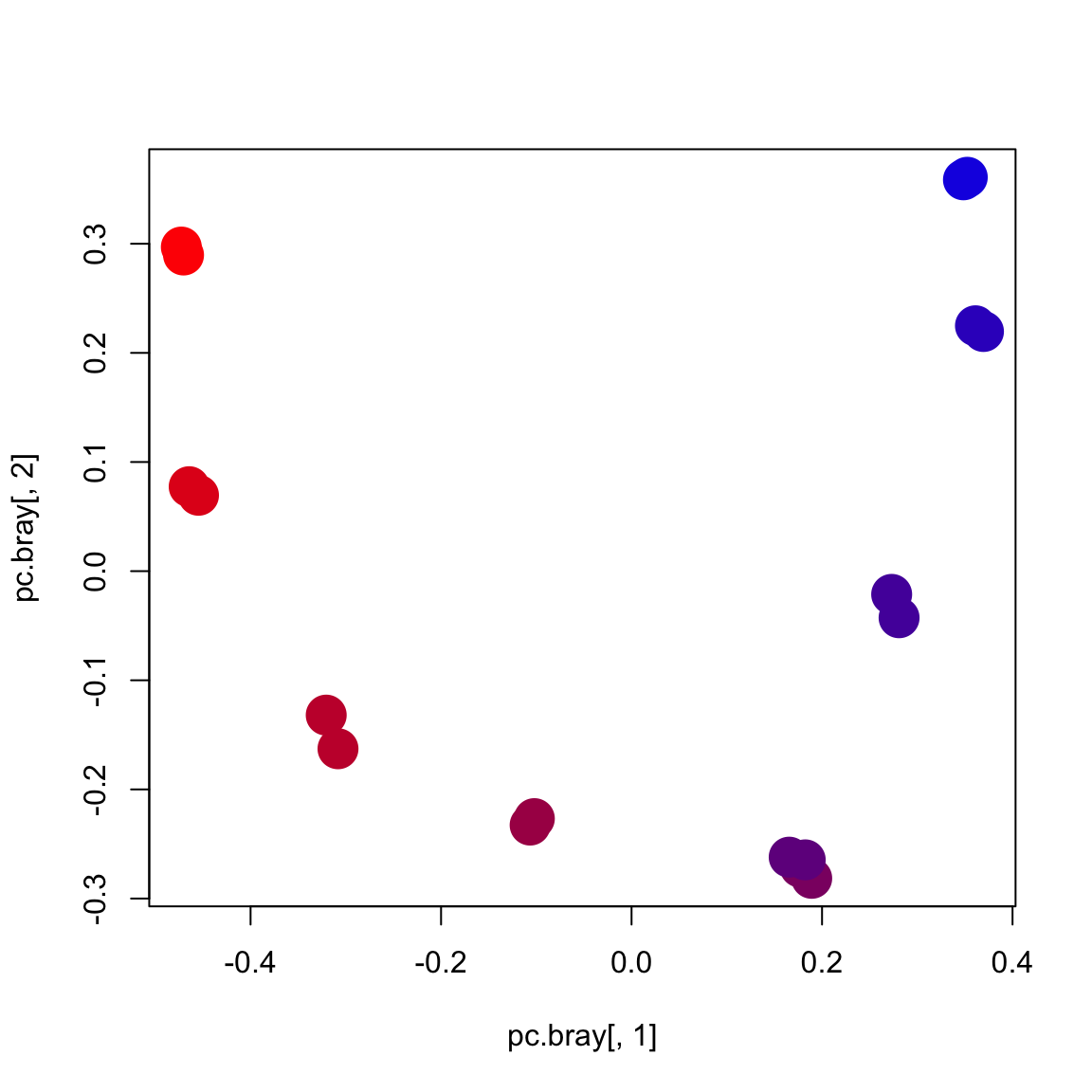All of the code in this page is meant to be run in `R` unless otherwise specified.

## Load data and calculate distance metrics. For more explanations of these commands see Beta diversity

``library('biom')``
``## Loading required package: methods``
``library('vegan')``
``## Warning: package 'vegan' was built under R version 3.2.3``
``## Loading required package: permute``
``## Warning: package 'permute' was built under R version 3.2.3``
``## Loading required package: lattice``
``## This is vegan 2.3-3``
``````# load biom file

# Extract data matrix (OTU counts) from biom table
otus <- as.matrix(biom_data(otus.biom))

# transpose so that rows are samples and columns are OTUs
otus <- t(otus)

# convert OTU counts to relative abundances
otus <- sweep(otus, 1, rowSums(otus),'/')

# find the overlapping samples
common.ids <- intersect(rownames(map), rownames(otus))

# get just the overlapping samples
otus <- otus[common.ids,]
map <- map[common.ids,]

# get Euclidean distance
d.euc <- dist(otus)

# get Bray-Curtis distances (default for Vegan)
d.bray <- vegdist(otus)

# get Chi-square distances using vegan command
# we will extract chi-square distances from correspondence analysis
my.ca <- cca(otus)
d.chisq <- as.matrix(dist(my.ca\$CA\$u[,1:2]))``````

## Ordination: PCA

Outside the field of ecology people often use principal components analysis (PCA). PCA is equivalent to calculating Euclidean distances on the data table, and then doing principal coordinates analysis (PCoA). The benefit of PCoA is that it allows us to use any distance metric, and not just Euclidean distances.

We will calculate PCA just for demonstration purposes. In practice, Euclidean distance is generally not a good distance metric to use on community ecology data. PCA will only work if there are more samples than features, so we will calculate it using just the top 10 OTUs.

``````# get the indices of the 10 dominant OTUs
otu.ix <- order(colMeans(otus),decreasing=TRUE)[1:10]

# PCA on the subset including 10 OTUs
pca.otus <- princomp(otus[,otu.ix])\$scores

# For comparison, do PCoA on Euclidean distances
pc.euc <- cmdscale(dist(otus[,otu.ix]))

# plot the PC1 scores for PCA and PCoA
# note: we might have to flip one axis because the directionality is arbitrary
# these are perfectly correlated
plot(pc.euc[,1], pca.otus[,1])``````## Ordination: NMDS

Another common approach is non-metric multidimensional scaling (MDS or NMDS). We can do this using the Vegan package. We will apply this to Bray-Curtis distances and plot the ordination colored by physical sample depth in the microbial mat.

``````# NMDS using Vegan package
mds.bray <- metaMDS(otus)\$points``````
``````## Run 0 stress 0.02317516
## Run 1 stress 0.0218455
## ... New best solution
## ... procrustes: rmse 0.0339146  max resid 0.07164408
## Run 2 stress 0.04503052
## Run 3 stress 0.04503124
## Run 4 stress 0.02330075
## Run 5 stress 0.02322204
## Run 6 stress 0.02184519
## ... New best solution
## ... procrustes: rmse 7.821081e-05  max resid 0.0001562467
## *** Solution reached``````
``````# makes a gradient from red to blue
my.colors <- colorRampPalette(c('red','blue'))(10)

layer <- map[,'LAYER']
plot(mds.bray[,1], mds.bray[,2], col=my.colors[layer], cex=3, pch=16)``````## Ordination: PCoA

NMDS seems to work fine at recovering a smooth gradient along PC1. Let us compare to PCoA on the same Bray-Curtis distances.

``````# Run PCoA (not PCA)
pc.bray <- cmdscale(d.bray,k=2)
plot(pc.bray[,1], pc.bray[,2], col=my.colors[layer], cex=3, pch=16)``````In general, PCoA and NMDS tend to give similar results. PCoA is more common in microbiome analysis.

## Biplots

One benefit of PCA over PCoA is that it automatically provides “loadings” for the features (OTUs/taxa/genes) along each axis, which can help visualize which features are driving the positions of samples along the principal axes of variation. This kind of plot is called a “biplot”. Fortunately there are ways to produce biplots using PCoA. The way that we make biplots with PCoA is to plot each species as a weighted average of the positions of the samples in which it is present. The weights are the relative abundances of that species in the samples.

``````# First get a normalized version of the OTU table
# where each OTU's relative abundances sum to 1
otus.norm <- sweep(otus,2,colSums(otus),'/')

# use matrix multiplication to calculated weighted average of each taxon along each axis
wa <- t(otus.norm) %*% pc.bray

# plot the PCoA sample scores
plot(pc.bray[,1], pc.bray[,2], col=my.colors[layer], cex=3, pch=16)

# add points and labels for the weighted average positions of the top 10 dominant OTUs
points(wa[otu.ix,1],wa[otu.ix,2],cex=2,pch=1)
text(wa[otu.ix,1],wa[otu.ix,2],colnames(otus)[otu.ix],pos=1, cex=.5)``````